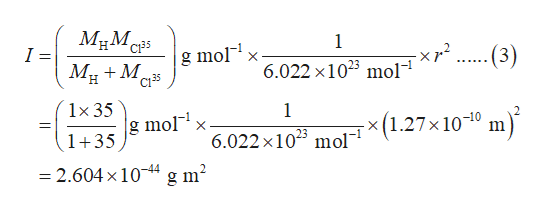# What is J_max in rotational Boltzman distribution, and calculate J_max for HCl at 1000K

Question
8 views

What is J_max in rotational Boltzman distribution, and calculate J_max for HCl at 1000K

check_circle

star
star
star
star
star
1 Rating
Step 1

The moment of inertia is calculated as shown in equation (1) where μ is the reduced mass and r is the bond length.

Step 2

Reduced mass for the HCl molecule is calculated as shown in equation (2) where MH and MCl(35) are molar masses of hydrogen and chlorine.

Step 3

The moment of inertia (I) in gm2 for the HCl is calculated as shown in equation (3) where MH and MCl(35) are molar masses of hydrogen and chlorine, and r is the bond length. The values for r,...help_outlineImage TranscriptioncloseМЕМ, I = 1 C135 mol C135 -(3) 6.022 х1023 mol- М. + M. н 1x 35 1 mol x(1.27х10-10 m 23 6.022 х10° mol 1+35 — 2.604 х10-44 g m? fullscreen

### Want to see the full answer?

See Solution

#### Want to see this answer and more?

Solutions are written by subject experts who are available 24/7. Questions are typically answered within 1 hour.*

See Solution
*Response times may vary by subject and question.
Tagged in

### Chemistry